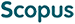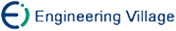王建卫, 郝忠孝. 概率XML文件树结点概率的查询算法[J]. 计算机研究与发展, 2012, 49(4): 785-794.
 引用本文: 王建卫, 郝忠孝. 概率XML文件树结点概率的查询算法[J]. 计算机研究与发展, 2012, 49(4): 785-794.Wang Jianwei, Hao Zhongxiao. Node Probability Query Algorithm in Probabilistic XML Document Tree[J]. Journal of Computer Research and Development, 2012, 49(4): 785-794.
 Citation: Wang Jianwei, Hao Zhongxiao. Node Probability Query Algorithm in Probabilistic XML Document Tree[J]. Journal of Computer Research and Development, 2012, 49(4): 785-794.## Node Probability Query Algorithm in Probabilistic XML Document Tree

• 摘要: 概率XML文件是概率数据的网络数据交换和表示标准，元素取值及其概率的查询与计算是概率XML文件的重要研究内容.概率XML文件树是一种有效的概率XML文件的数据模型，定义了概率XML文件树的基本路径和扩展路径，提出了根据可能世界原理将概率XML文件树分解为普通子XML树的集合的算法，根据路径分析原理将概率XML文件树分解为子概率XML树的集合的算法和相应的查询与计算结点及结点集合概率的算法，并通过实验进行了比较分析.实验结果表明：这两种方法是有效的；与前一种方法比较，后一种方法适合较大的概率XML文件树、结点及结点集合的概率的查询，计算过程较简单.

Abstract: Because probabilistic XML document is the network data exchange and representation standard of probabilistic data, query and computation method of the element or elements and the probability is a main research content. Probabilistic XML document tree is an effective data model of probabilistic XML document, so this paper presents two kinds of query algorithms based on the definitions of basic path expression and extended path expression. One is that the probabilistic XML tree is firstly decomposed into the set of ordinary XML subtrees based on possible world principle and then the probability query algorithm of a single node or some nodes is designed. The other is that the probabilistic XML tree is firstly decomposed into the set of probabilistic XML subtrees based on the path analysis principle and then the probability query algorithm is also designed. The two algorithms are compared and analyzed through the experiments and the results show that they are effective. Compared with the first method, the second one fits the larger probabilistic XML document tree, and the quering and computeation of node probability are easier./下载:  全尺寸图片 幻灯片
• 分享
• 用微信扫码二维码

分享至好友和朋友圈